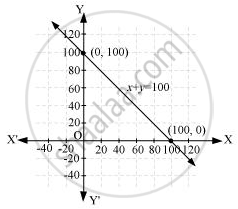# Yamini and Fatima, two students of Class IX of a school, together contributed Rs. 100 towards the Prime Minister’s Relief Fund to help the earthquake victims. Write a linear equation which satisfies this data. - Mathematics

Yamini and Fatima, two students of Class IX of a school, together contributed Rs. 100 towards the Prime Minister’s Relief Fund to help the earthquake victims. Write a linear equation which satisfies this data. (You may take their contributions as Rs. x and Rs. y.) Draw the graph of the same.

#### Solution

Let the amount that Yamini and Fatima contributed be x and y respectively towards the Prime Minister’s Relief fund.

Amount contributed by Yamini + Amount contributed by Fatima = 100

y = 100

It can be observed that (100, 0) and (0, 100) satisfy the above equation. Therefore, these are the solutions of the above equation. The graph is constructed as follows.Here, it can be seen that variable x and y are representing the amount contributed by Yamini and Fatima respectively and these quantities cannot be negative. Hence, only those values of x and y which are lying in the 1st quadrant will be considered.

Concept: Graph of a Linear Equation in Two Variables
Is there an error in this question or solution?
Chapter 4: Linear Equations in two Variables - Exercise 4.3 [Page 74]

#### APPEARS IN

NCERT Class 9 Maths
Chapter 4 Linear Equations in two Variables
Exercise 4.3 | Q 7 | Page 74

Share